# ROW function

The ROW function in Excel returns the row number of a reference. For example, =ROW(C4) returns 4. The ROW function can be quite useful.

1. The ROW function below returns 8.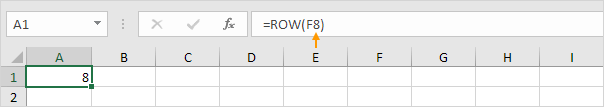2. If the reference is omitted, the ROW function returns the row number of the cell containing the ROW formula.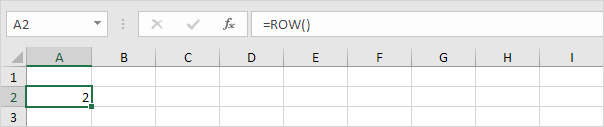3. To clearly see this, select cell A2, click on the lower right corner of cell A2, and drag the ROW function down to cell A9.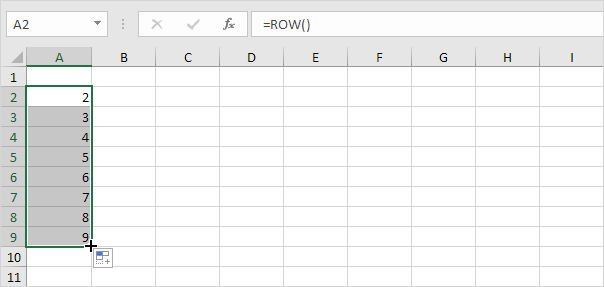4. If the reference is a range, the ROW function (entered in a single cell) returns the row number of the topmost row.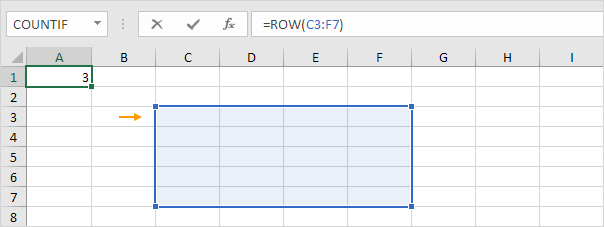Note: to return all 5 row numbers, first select the range A1:A5 (or another range of the same size), enter =ROW(C3:F7) and finish by pressing CTRL + SHIFT + ENTER. If you have Excel 365 or Excel 2021, simply select cell A1, enter =ROW(C3:F7) and press Enter.

5. Don't confuse the ROW function with the ROWS function. The ROWS function counts the number of rows in a range.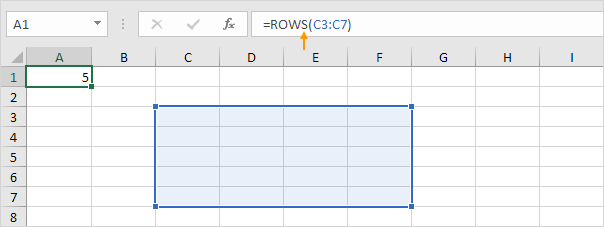Why using the ROW function in Excel?

6. For example, you can use the ROW function to sum every nth row in Excel (in the example below, every 3rd row).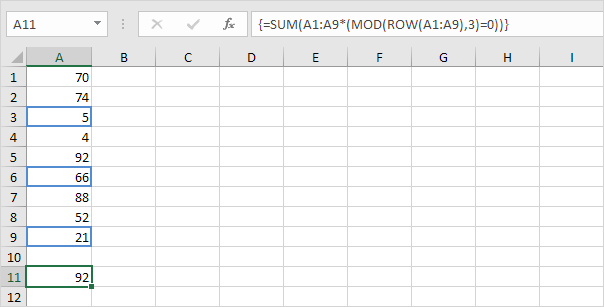Tip: use ROW, MOD and conditional formatting to shade alternate rows in Excel.

Go to Next Chapter: Formulas and Functions